# RD Sharma Solutions for Class 9 Maths Chapter 17 Construction Exercise 17.2

Exercise 17.2 of RD Sharma textbook solutions are given here. This exercise is based on construction of the bisector of a given angle. The questions are also according to the syllabus and guidelines of CCE and Central Board of Secondary Education. Exercise 17.2 of RD Sharma Class 9 Chapter 17 helps students to learn how to construct different angles with the help of ruler and compass only. Click on the link below to get your pdf now.

## Download PDF of RD Sharma Solutions for Class 9 Maths Chapter 17 Construction Exercise 17.2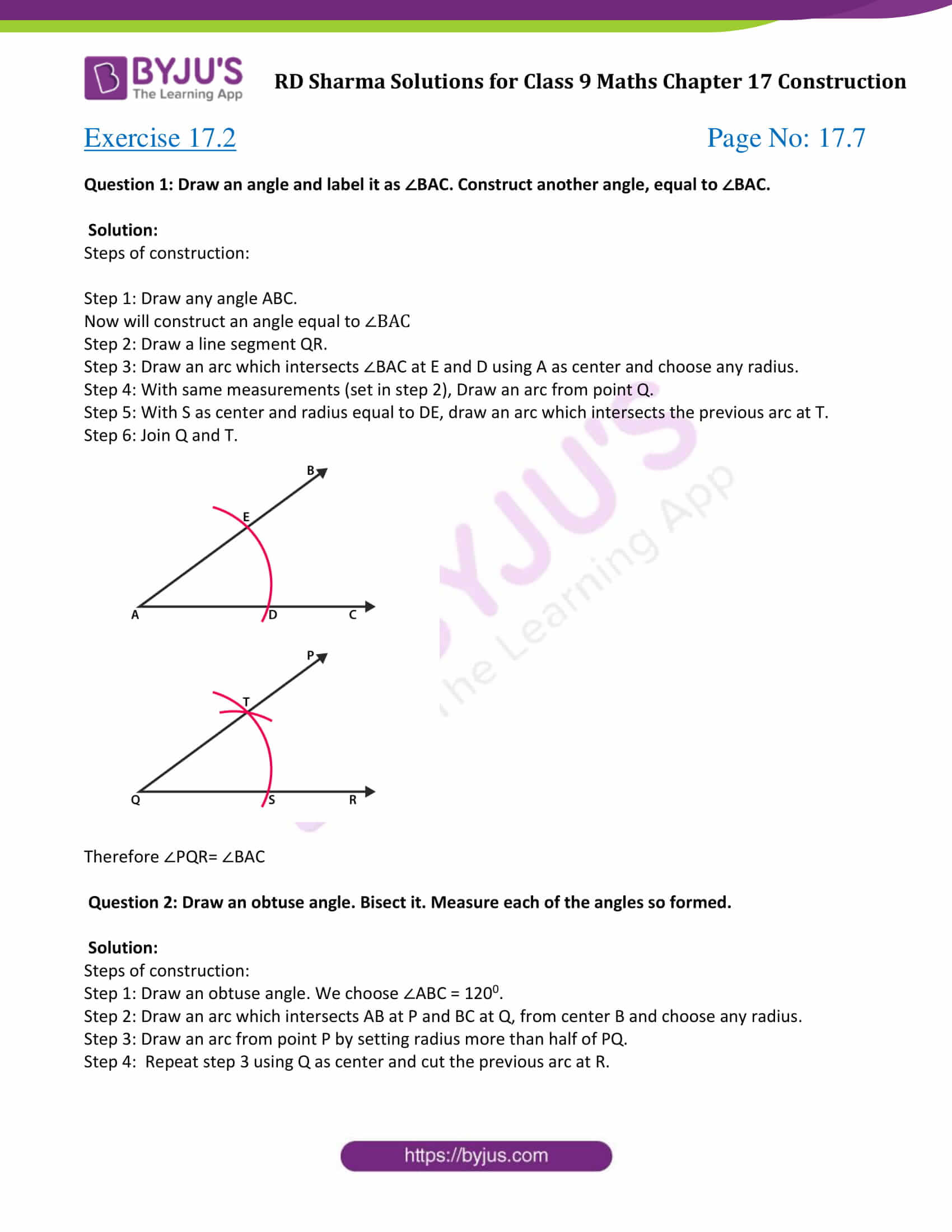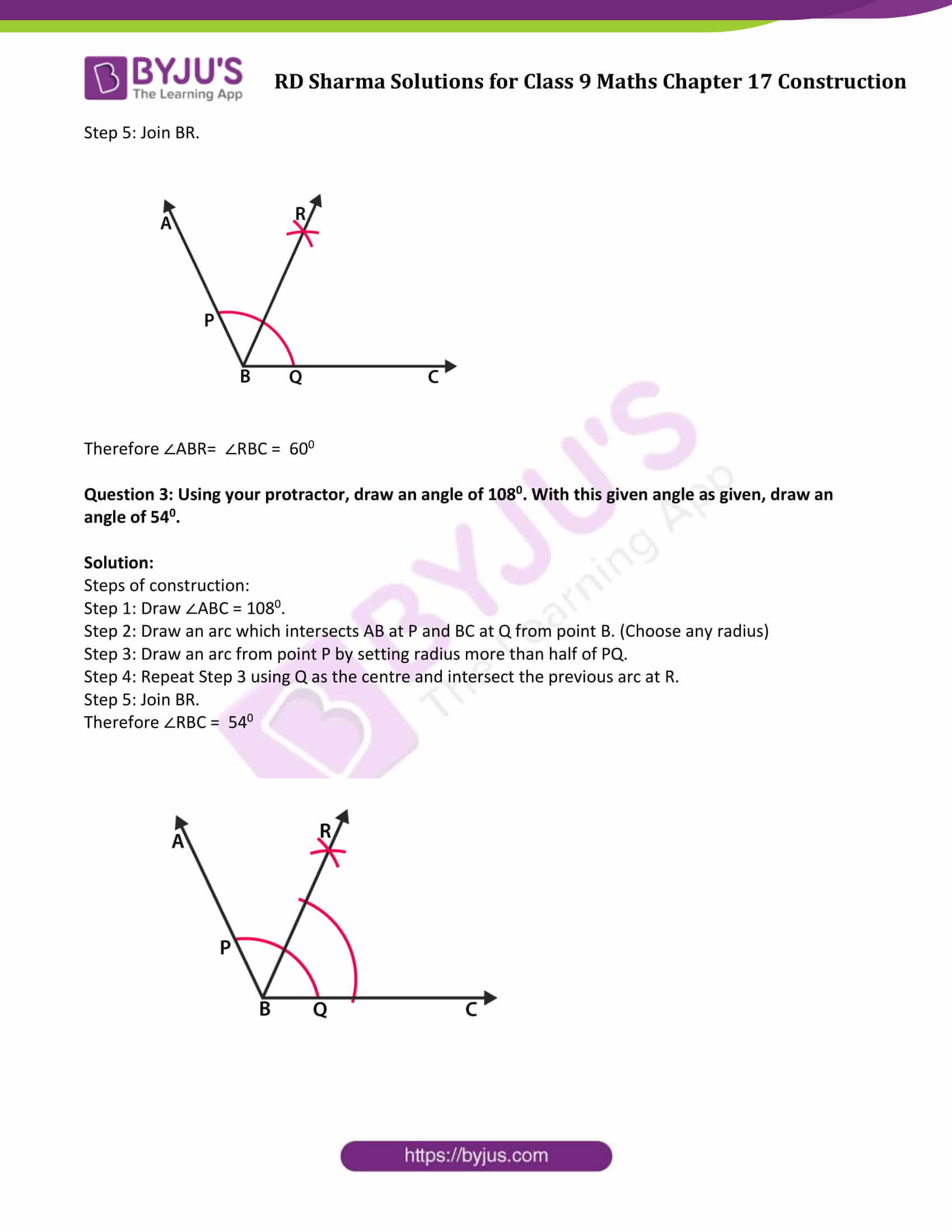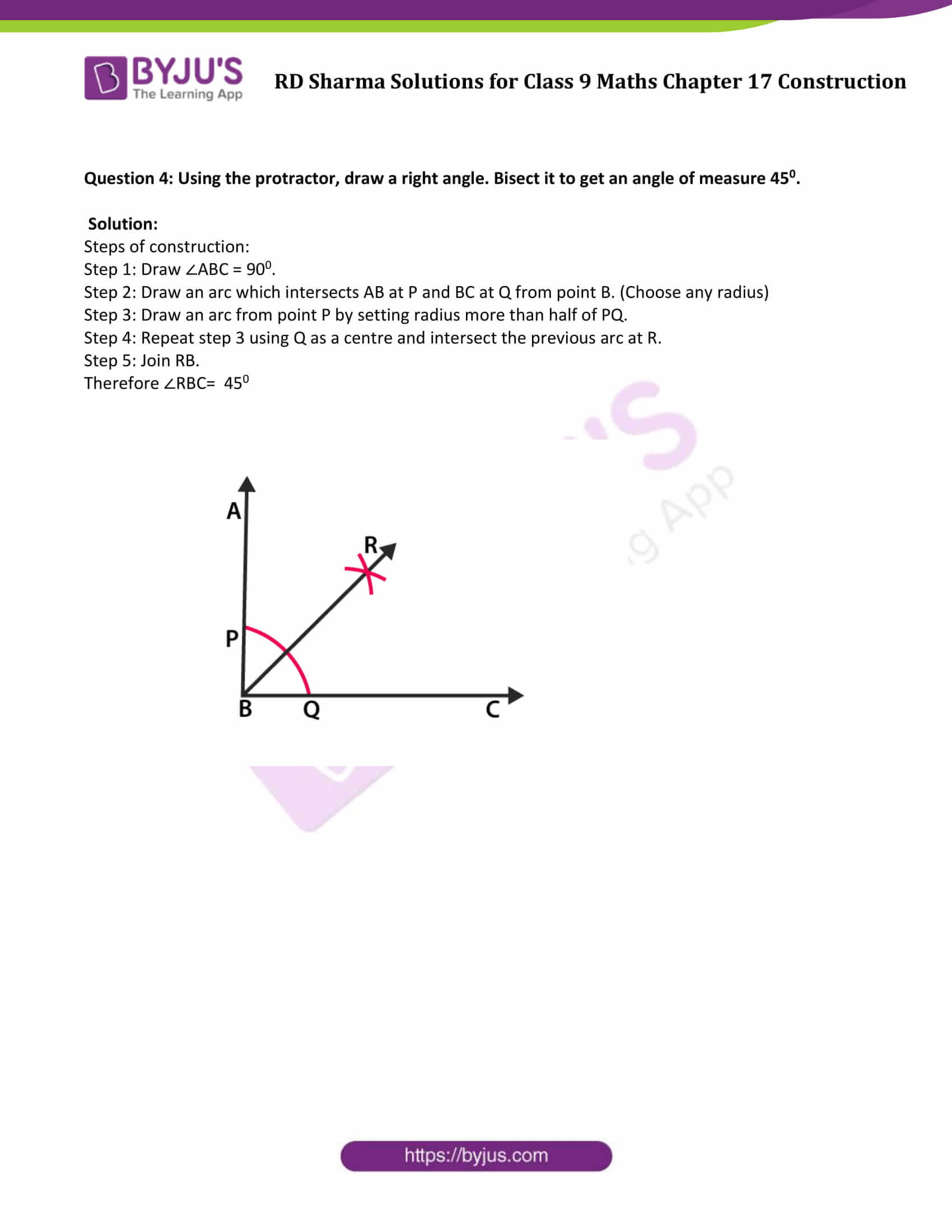### Access Answers to Maths RD Sharma Solutions for Class 9 Chapter 17 Construction Exercise 17.2 Page number 17.7

Question 1: Draw an angle and label it as ∠BAC. Construct another angle, equal to ∠BAC.

Solution:

Steps of construction:

Step 1: Draw any angle ABC.

Now will construct an angle equal to ∠BAC

Step 2: Draw a line segment QR.

Step 3: Draw an arc which intersects ∠BAC at E and D using A as center and choose any radius.

Step 4: With same measurements (set in step 2), Draw an arc from point Q.

Step 5: With S as center and radius equal to DE, draw an arc which intersects the previous arc at T.

Step 6: Join Q and T.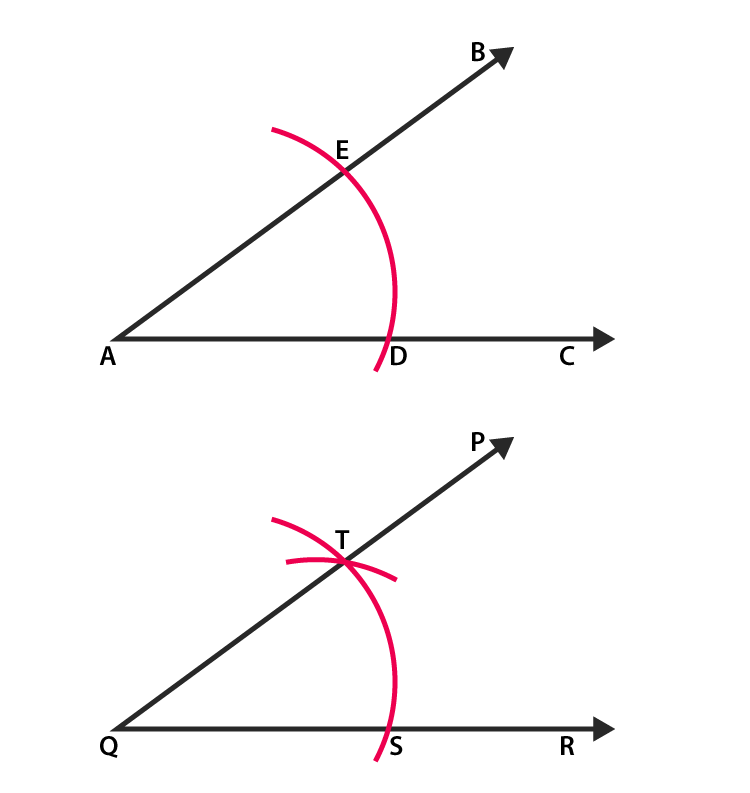Therefore ∠PQR= ∠BAC

Question 2: Draw an obtuse angle. Bisect it. Measure each of the angles so formed.

Solution:

Steps of construction:

Step 1: Draw an obtuse angle. We choose ∠ABC = 1200.

Step 2: Draw an arc which intersects AB at P and BC at Q, from center B and choose any radius.

Step 3: Draw an arc from point P by setting radius more than half of PQ.

Step 4: Repeat step 3 using Q as center and cut the previous arc at R.

Step 5: Join BR.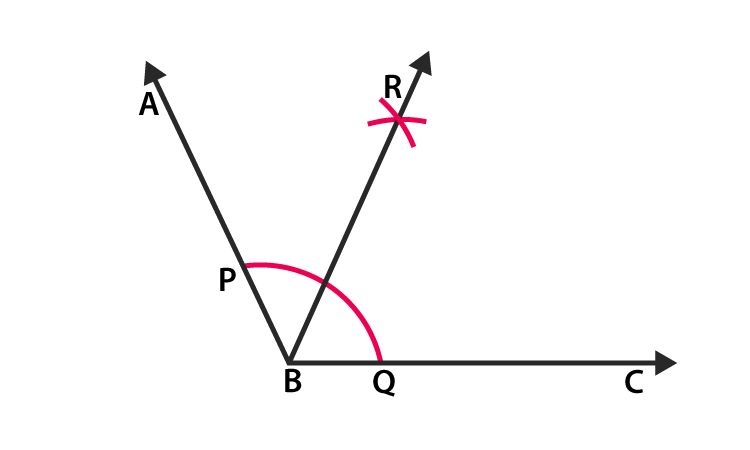Therefore ∠ABR= ∠RBC = 600

Question 3: Using your protractor, draw an angle of 1080. With this given angle as given, draw an angle of 540.

Solution:

Steps of construction:

Step 1: Draw ∠ABC = 1080.

Step 2: Draw an arc which intersects AB at P and BC at Q from point B. (Choose any radius)

Step 3: Draw an arc from point P by setting radius more than half of PQ.

Step 4: Repeat Step 3 using Q as the centre and intersect the previous arc at R.

Step 5: Join BR.

Therefore ∠RBC = 540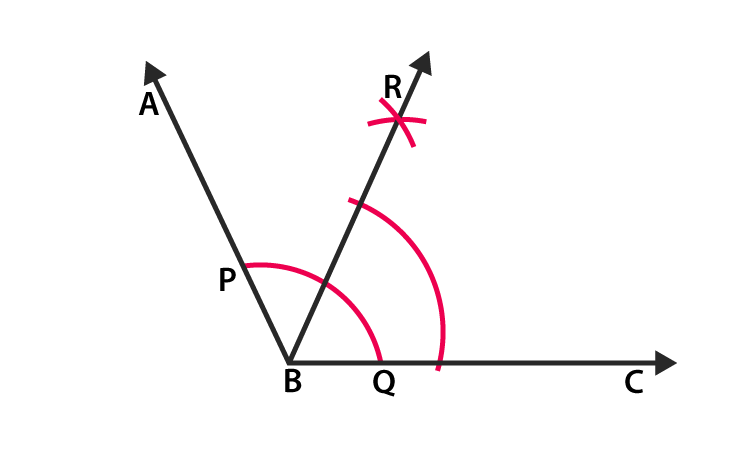Question 4: Using the protractor, draw a right angle. Bisect it to get an angle of measure 450.

Solution:

Steps of construction:

Step 1: Draw ∠ABC = 900.

Step 2: Draw an arc which intersects AB at P and BC at Q from point B. (Choose any radius)

Step 3: Draw an arc from point P by setting radius more than half of PQ.

Step 4: Repeat step 3 using Q as a centre and intersect the previous arc at R.

Step 5: Join RB.

Therefore ∠RBC= 450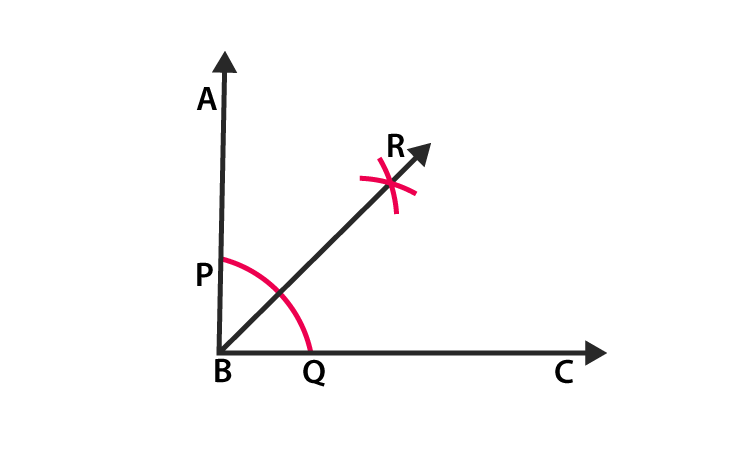## RD Sharma Solutions for Class 9 Maths Chapter 17 Construction Exercise 17.2

RD Sharma Solutions Class 9 Maths Chapter 17 Construction Exercise 17.2 is based on the following topics:

• Construction of the Bisector of a Given Angle
• Construction of some Standard Angles – 300, 600, 900, 450 and 1200

In this exercise, students will learn how to construct angles of 300, 600, 900, 450 and 1200 with the help of ruler and compass only.